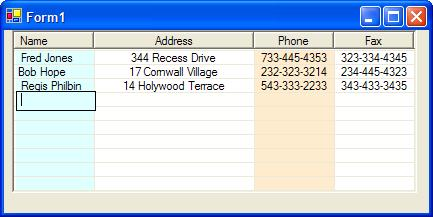# Grid View Control V - Sizing and Tabbing in the GridView

(* note when running this application, you may need to add the reference to the Microsoft Excel 11.0 Object Library)Figure 1 - GridView Control Sized to fit Columns and Rows

Introduction

Coding to Fit the Grid

The GridView was sized by breaking down the methods into SizeToFitRows and SizeToFitColumns.  These methods simply call set bounds on the GridView control to make all the rows and columns visible respectively.  Both methods use the SetBounds method of the control.  The methods call CalculateHeight and CalculateWidth in order to determine the width and height of the total columns and total rows.

int CalculateHeight()
{
if (NumberOfRows == 0)
return 0; // precondition
// calculate height based on the number of rows and the height of an item in the row
int height = (this.NumberOfRows + 2) * listView1.Items[this.NumberOfRows -]
.Bounds.Height;
return height-5;
}
int CalculateWidth()
{
if (NumColumns == 0)
return 0; // precondition
//width is based on the sum of all the column widths
int width = SumColumnWidths();
return width + 5;
}
public void SizeToFitRows()
{
// set the bounds of the grid based on the total height of the rows
SetBounds(this.Left, this.Top, this.Width, CalculateHeight());
}
public void SizeToFitColumns()
{
// set the bounds of the grid based on the total width of the columns
SetBounds(this.Left, this.Top, CalculateWidth(), this.Height);
}
public void SizeToFitAll()
{
SizeToFitRows();
SizeToFitColumns();
}

Processing the Tab

It took me a bit to figure out how to capture the tab key in code because the tab key is a command key so it will not necessarily be trapped in the event handlers for KeyPress or KeyDown.  I thought maybe I had to capture the tab in the WndProc but this proved fruitless as well.  It turns out that the tab key event is trapped in an override called ProcessCmdKey.  This method actually gets called when you press the tab key when inside an edit box.  The code for trapping the tab key press and tabbing the edit field in the GridView is shown below.  Note you can also test for modifier keys such as CTRL and ALT by OR'ing the keyData with the command key in question.

protected override bool ProcessCmdKey(ref Message msg, Keys keyData)
{
// check if the message is a keydown or a system key down
if ((msg.Msg == WM_KEYDOWN) || (msg.Msg == WM_SYSKEYDOWN))
{
switch (keyData)
{
case (Keys.Tab | Keys.Control): // shift the edit field left
MoveCellTab(false);
break;
case Keys.Tab: // shift the edit field right
MoveCellTab(true);
break;
default:
break;
}
}
// handle the default behavior of the command key
return base.ProcessCmdKey(ref msg, keyData);
}

Previous GridView Articles